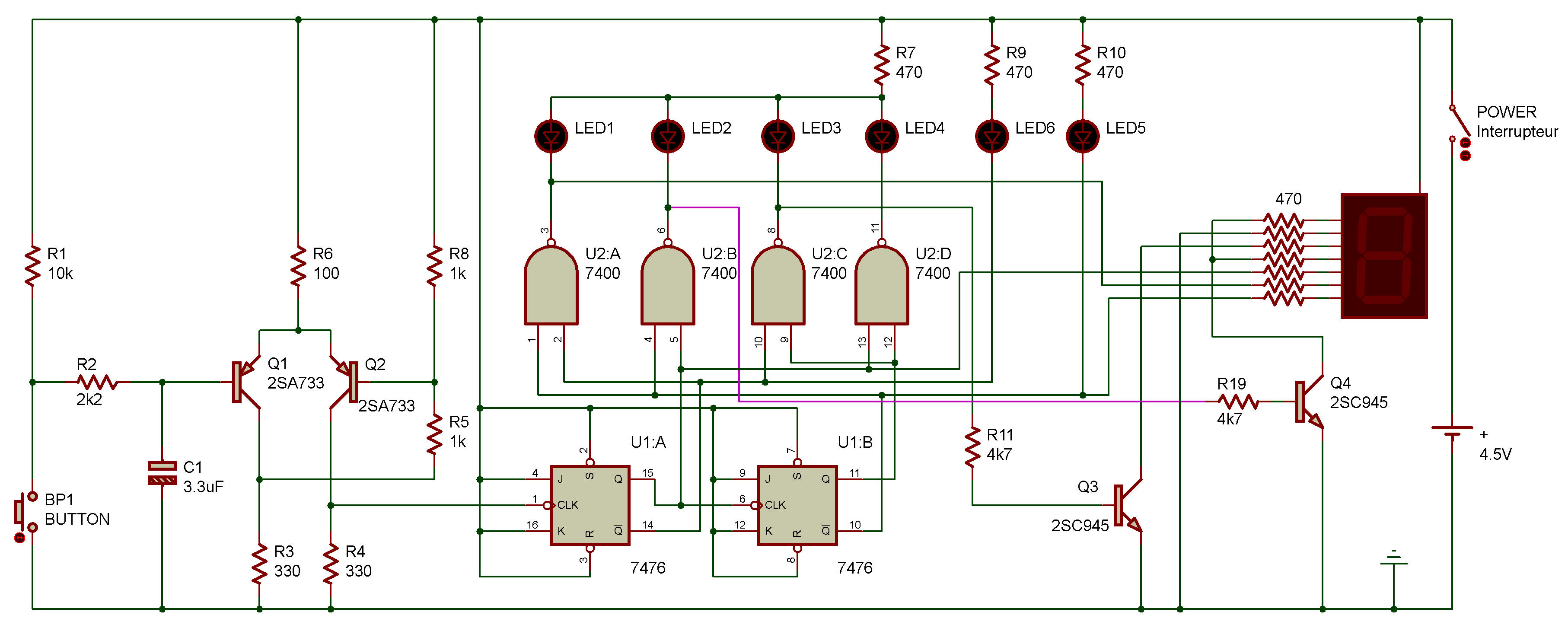Number displays
Electronic
7400, 7476Ever heard of binary numbers?

They're numbers made up of only Os and Is. This Project will count the presses of the Key and display the results in binary and decimal (1, 2, 3, etc.) form.

You can see from the schematic that this Project uses a divide by four counter and a line decoder. Each time you press the Key, you input a clock signal and cause the counter to advance by one.

When you finish building this Project, put power to ON. Press the Key slowly.

You'll see the Display show the numerals 0, 1, 2 and 3. LEDs 1 through 4 will light up in turn as the Key is pressed. But look at LEDs 5 and 6 - why are they lighting up like they do?

LEDs 5 and 6 are displaying binary numbers. Suppose 0 represents an LED that is off and 1 represents an LED that is lit. As you press the Key, here's what you'll see LEDs 5 and 6 do:

(0 0) (0 1) (1 0) (1 1) (0 0)

That illustrates how the binary number system goes. (Remember? Let's review ...)

0 is 0 just like in our decimal system, and 1 is also 1.

But what happens when we reach 2?

In our decimal system, we use the digits 0 through 9 to make up all numbers. When we reach 9 in the decimal system, we go to 10.

The same thing happens in the binary system. "10" represents 2, "11" represents 3 ... and "100" represents 4.

Since our counter only counts 0, 1, 2 and 3, "11" is the largest binary number displayed by this circuit. Ask your math teacher to recommend some books about the binary number system.
See Binary Number System

We've already mentioned that binary numbers are used to store numbers in computers. Sometimes you'll see the words octal and hexadecimal used to describe the numbers used in microcomputer systems. Try looking up the meanings for those two words in a dictionary.

Recherche personnalisée